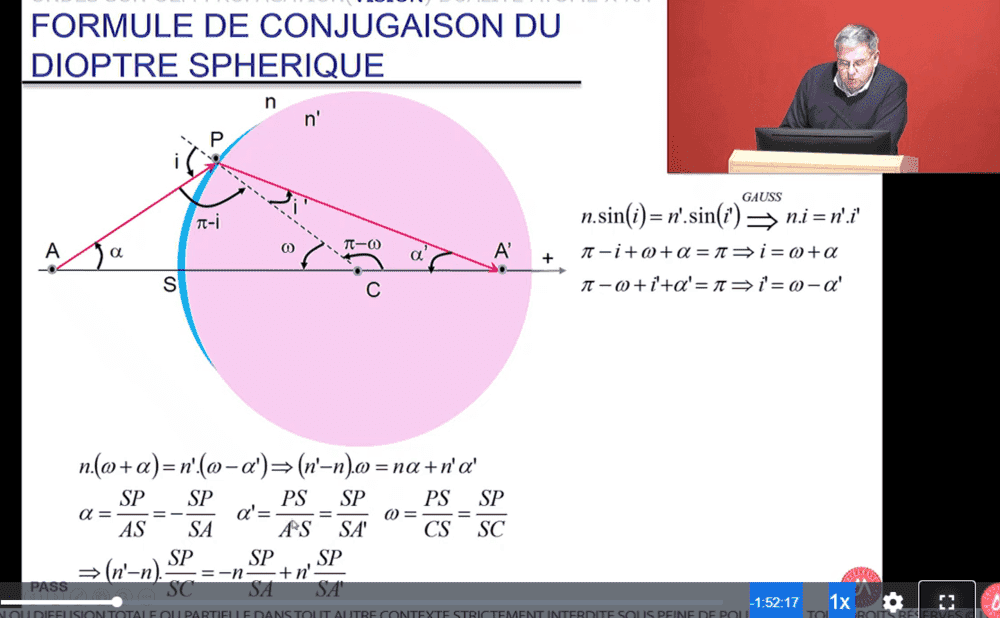# Spherical diopter formula

duchuy
Homework Statement:
I can't seem to understand the relation
Relevant Equations:
angle = length / length
Hi,
I don't understand how the professor managed to determine the values of alpha, alpha' and omega. What is the formula tha´t is applied to determine alpha = SP / AS and so on... knowing that alpha is a really small angle. CheersHomework Helper
2022 Award
You need to ask specific questions one at a time as you try to work through this. You could start by saying in specific detail what you wish to know when we are finished here.
The typical way to define an angle (in radians) is ?

duchuy
You need to ask specific questions one at a time as you try to work through this. You could start by saying in specific detail what you wish to know when we are finished here.
Thanks for your reply, would you then mind explaining me how the professor managed to determine that alpha = SP / AS? I did try to use trigonometric formulas for this but the results were nowhere near SP / AS. Thanks a lot.

duchuy
I don't really understand... PAC which is the alpha angle doesn't have A as the center of the circle and SP is the length of the the straight line SP not the curvature SP. Or in this case is SP referring to the length of the curvature? That would make sense for the omega angle but still not for alpha no?
Thanks